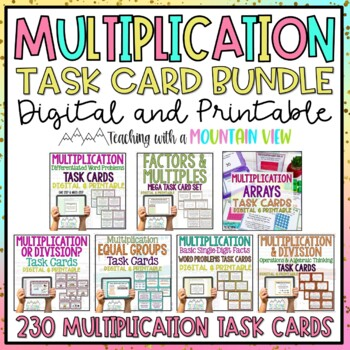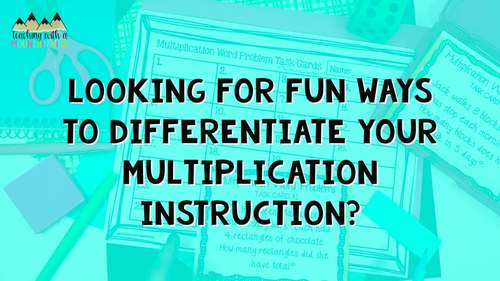Rated 4.83 out of 5, based on 3820 reviews
3.8k Ratings;
3rd - 5th
Subjects
Resource Type
Standards
Formats Included
• Zip
•Google Apps™
Pages
76 pages
\$17.65
List Price:
\$19.61
You Save:
\$1.96
Bundle
\$17.65
List Price:
\$19.61
You Save:
\$1.96
Bundle
Report this resource to TPTThis bundle contains one or more resources with Google apps (e.g. docs, slides, etc.).

#### What educators are saying

These task cards were perfect to use during small group instruction with my special needs third graders!
I used this in a small group intervention/reteach lesson with struggling 4th graders. These task cards provided the perfect practice that we needed.

#### Products in this Bundle (6)

showing 1-5 of 6 products

##### Also included in
1. An absolute must-have, MASSIVE math task card bundle. I have been asked over and over to bundle all of my task card collections together, and here it is! There are over 2,000 Math Task Cards in this huge discounted bundle of all my bundles (PLUS NINE sets of previously unbundled task cards!) The c
Price \$159.26Original Price \$176.95Save \$17.69
2. Are you looking to teach (and differentiate) multiplication to students in third, fourth, or fifth grades? This is the ultimate collection of hands-on resources you need! This set of hundreds of math task cards covers a wide variety of multiplication skills!The following 12 Sets of Multiplication
Price \$24.99Original Price \$31.18Save \$6.19
3. Get ready to take engagement to the next level with these multiplication and division activities that are just perfect for 3rd grade. Are you learning about multiplication and division strategies? Do you need activities that are SO easy to prep and teach? This is the bundle for you!Help your student
Price \$39.99Original Price \$62.27Save \$22.28

### Description

This set of 230 multiplication task cards provides ample opportunities for your students to practice multiplication (and a few division) skills.

ALL SETS INCLUDE A DIGITAL VERSION in either Google Forms or Google Slides.

The Following Individual Task Card Sets are Included:

• Multiplication Word Problem Task Cards Common Core, One step and Multi Step: A set of 32 word problem task cards for your Common Core classroom learning multiplication! The first 16 cards are one step, one by two, three or four digit multiplication. The second 16 cards are multi-step, one by two, three, or four digit multiplication.

• Multiplication ARRAY Task Cards: A set of 32 Multiplication Array Task Cards aligned to Common Core Standards. Varying tasks are included! (DIGITAL included)

• Multiplication or Division Task Cards: A complete set of 32 basic multiplication and division task cards. You can differentiate instruction by "scaffolding" the lesson...start out by having students simply determine whether you use division or multiplication to solve the problem, then move on to writing the equation, drawing a picture, solving, etc. (DIGITAL included)

• Multiplication and Division (Writing Equations & Solving Task Cards) : A set of 40 task cards fully aligned to 3rd grade (perfect for differentiation for 2nd and 4th grade) Common Core Standards for writing and solving multiplication and division equations. The final 8 cards include all four operations so that the cards are fully and completely aligned to the Operations and Algebraic Thinking Common Core Standards.

• Multiplication Equal Groups Task Cards : 32 task cards to practice multiplying equal groups! (DIGITAL included)

• Basic Multiplication Word Problem Task Cards : 32 task cards to practice basic multiplication facts in word problem format. All are one digit by one digit multiplication and one step.

Answer Keys and Recording Sheets Included for All!

Are you looking for even MORE multiplication resources?

Resort Report: A Multi-Digit Multiplication Project

Multi-Digit Multiplication Error Analysis

⭐⭐⭐⭐⭐ “STOP!!! LOOK NO FURTHER! As a 30 year veteran teacher of 3rd grade, I rate this **** 4 stars. The quality presented here is far and above that of similar products by any other seller. SIMPLY SUPERB!" - Kyle A.

⭐⭐⭐⭐⭐ “My students love doing these task cards! We have used both the digital option and the print option and both are engaging and challenging for them as we work on the skills. Thank you for all the hard work!" - Janet P.

⭐⭐⭐⭐⭐"I love these task cards! There are so many ways to use them in the classroom. I love placing them around the room and having students work at their own pace - great time to help other students or work with small groups also!" - Wild Wonders Teaching

Are you looking for Inspiration, Community, and Teaching Support? Join my private Facebook Group! We collaborate, share ideas, and support one another every day. Click HERE to join Inspired in Upper Elementary.

Total Pages
76 pages
Included
Teaching Duration
N/A
Report this resource to TPT
Reported resources will be reviewed by our team. Report this resource to let us know if this resource violates TPT’s content guidelines.

### Standards

to see state-specific standards (only available in the US).
Interpret products of whole numbers, e.g., interpret 5 × 7 as the total number of objects in 5 groups of 7 objects each. For example, describe a context in which a total number of objects can be expressed as 5 × 7.
Interpret whole-number quotients of whole numbers, e.g., interpret 56 ÷ 8 as the number of objects in each share when 56 objects are partitioned equally into 8 shares, or as a number of shares when 56 objects are partitioned into equal shares of 8 objects each. For example, describe a context in which a number of shares or a number of groups can be expressed as 56 ÷ 8.
Use multiplication and division within 100 to solve word problems in situations involving equal groups, arrays, and measurement quantities, e.g., by using drawings and equations with a symbol for the unknown number to represent the problem.
Determine the unknown whole number in a multiplication or division equation relating three whole numbers. For example, determine the unknown number that makes the equation true in each of the equations 8 × ? = 48, 5 = __ ÷ 3, 6 × 6 = ?.
Apply properties of operations as strategies to multiply and divide. Examples: If 6 × 4 = 24 is known, then 4 × 6 = 24 is also known. (Commutative property of multiplication.) 3 × 5 × 2 can be found by 3 × 5 = 15, then 15 × 2 = 30, or by 5 × 2 = 10, then 3 × 10 = 30. (Associative property of multiplication.) Knowing that 8 × 5 = 40 and 8 × 2 = 16, one can find 8 × 7 as 8 × (5 + 2) = (8 × 5) + (8 × 2) = 40 + 16 = 56. (Distributive property.)